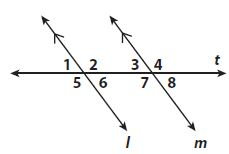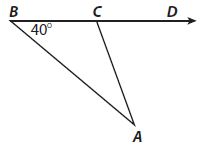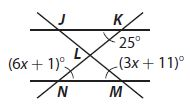Homework Explained - Math Practice 101Dear guest, you are not a registered member. As a guest, you only have read-only access to our books, tests and other practice materials.

As a registered member you can:

Registration is free and doesn't require any type of payment information. Click here to Register.
Go to page:
Chapter 11:Angle Relationships in Parallel Lines and Triangles; Mixed Review

### Selected Response

Use the figure for Exercises 1 and 2.• Question 1

Which angle pair is a pair of alternate exterior angles?

• A. $$\angle5$$ and $$\angle6$$
• B. $$\angle6$$ and$$\angle7$$
• C. $$\angle5$$ and $$\angle4$$
• D. $$\angle5$$ and $$\angle2$$
• Question 2

Which of the following angles is not congruent to $$\angle$$3?

• A. $$\angle1$$
• B. $$\angle2$$
• C. $$\angle6$$
• D. $$\angle8$$
• Question 3

The measures, in degrees, of the three angles of a triangle are given by 2x + 1, 3x - 3, and 9x. What is the measure of the smallest angle?

• A. $$13^0$$
• B. $$27^0$$
• C. $$36^0$$
• D. $$117^0$$
• Question 4

Which is a possible measure of $$\angle DCA$$ in the triangle below?• A. $$36^0$$
• B. $$38^0$$
• C. $$40^0$$
• D $$70^0$$
• Question 5

Kaylee wrote in her dinosaur report that the Jurassic period was $$1.75 \times 10^8$$ years ago. What is this number written in standard form?

• A. 1,750,000
• B. 17,500,000
• C. 175,000,000
• D. 17,500,000,000
• Question 6

Given that y is proportional to x, what linear equation can you write if y is 16 when x is 20?

• A. y = 20x
• B. y =$$\frac{5}{4}$$ x
• C. y=$$\frac{4}{5}$$x
• D. y = 0.6x

• Question 7

Two transversals intersect two parallel lines as shown.a. What is the value of x?

•  x =
• Question 7

b. What is the measure of $$\angle LMN$$?

•  $$^°$$
• Question 7

c. What is the measure of $$\angle KLM$$?

•  $$\angle KLM =$$ $$^°$$
• Question 7

d. Which two triangles are similar? How do you know?

• Type below:

Yes, email page to my online tutor. (if you didn't add a tutor yet, you can add one here)